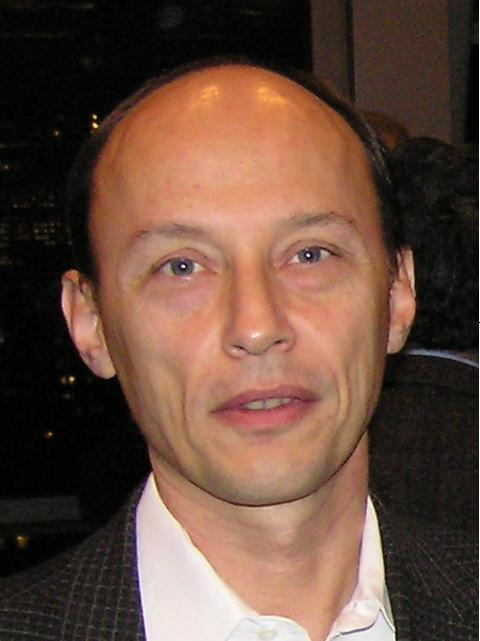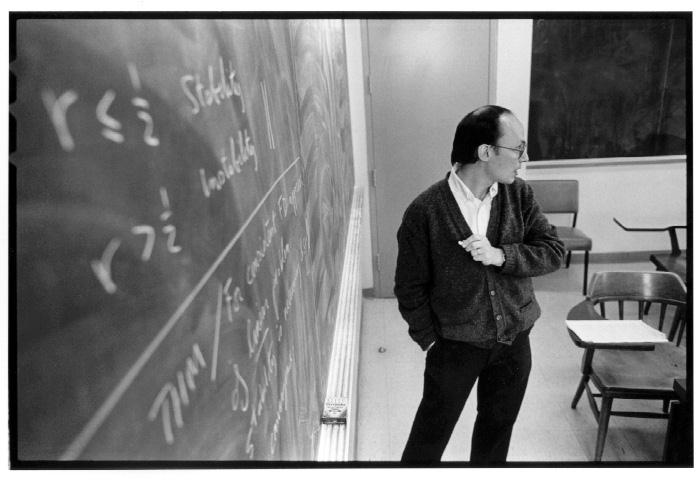## Leslie Greengard

Department of Mathematics
Courant Institute of Mathematical Sciences
New York University

### Contact

email: greengard at cims.nyu.edu
(212) 998-3306 (voice); (212) 995-4121 (fax)
Courant Institute, New York University
251 Mercer Street
New York, NY 10012

Numerical Methods II (Spring 2022)

### Research Interests

Scientific computing, fast algorithms, adaptive methods, integral equations, potential theory, electromagnetics, computational chemistry, computational biology. >Click for more information on the following projects:

### Selected Publications

• A Fast Algorithm for Particle Simulations
(with V. Rokhlin).  J. Comput. Phys. 73, 325 (1987).
• A Fast Adaptive Multipole Algorithm for Particle Simulations [pdf: 1,922 Kb]
(with J. Carrier and V. Rokhlin).   SIAM J. Sci. Stat. Comput. 9, 669 (1988).
• Potential Flow in Channels [pdf: 1,827 Kb]
SIAM J. Sci. Stat. Comput. 11, 603 (1990).
• A Fast Algorithm for the Evaluation of Heat Potentials [Preprint, pdf: 170 Kb]
(with J. Strain ).   published version: Comm. Pure Appl. Math. 43, 949-963 (1990).
• The Fast Gauss Transform [pdf: 1,372 Kb]
(with J. Strain).   SIAM J. Sci. Stat. Comput. 12, 79 (1991).
• Spectral Integration and Two-Point Boundary Value Problems [pdf: 898 Kb]
SIAM J. Numer. Anal. 28, 1071 (1991).
• Laplace's Equation and the Dirichlet-Neumann Map in Multiply-Connected Domains
(with A. Greenbaum, G. B. McFadden).  J. Comput. Phys. 105, 267 (1993).
• A Renormalization Method for the Evaluation of Lattice Sums
(with C. L. Berman).  J. Math. Phys. 35, 6036 (1994).
• A Fast Poisson Solver for Complex Geometries
(with A. McKenney, A. Mayo).  J. Comput. Phys. 118, 348 (1995).
• A Direct Adaptive Poisson Solver of Arbitrary Order Accuracy
(with J.-Y. Lee).  J. Comput. Phys. 125, 415 (1996).
• A Fast Adpative Numerical Method for Stiff Two-Point Boundary Value Problems [pdf: 1,039 Kb]
(with J. Y. Lee).   SIAM J. Sci. Comput. 18, 403 (1997).
• A Method of Images for the Evaluation of Electrostatic Fields in Systems of Closely Spaced Conducting Cylinders [pdf: 1,130 Kb]
(with H. Cheng).   SIAM J. Appl. Math. 58, 122 (1998).
• An Integral Equation Approach to the Incompressible Navier-Stokes Equations in Two Dimensions [pdf: 1,039 Kb]
(with M. C. Kropinski).   SIAM J. Sci. Comput. 20, 318 (1998).
• On the Numerical Evaluation of Elastostatic Fields in Locally Isotropic Two-Dimensional Composites
(with J. Helsing ).  J. Mech. Phys. Solids 46, 1441 (1998).
• A Fast Adaptive Multipole Algorithm in Three Dimensions
(with H. Cheng, V. Rokhlin).  J. Comput. Phys. 155, 468 (1999).
• An Integral Evolution Formula for the Wave Equation
(with B. Alpert, T. Hagstrom).  J. Comput. Phys. 162, 536 (2000).
• Spectral Approximation of the Free-Space Heat Kernel [pdf: 158 Kb]
(with P. Lin).  Appl. Comput. Harmonic Anal. 9, 83 (2000).
• Coulomb Interactions on Planar Structures: Inverting the Square Root of the Laplacian [pdf: 509 Kb]
(with Z. Gimbutas, M. Minion).   SIAM J. Sci. Comput. 22, 6 (2001).
• A New Fast-Multipole Accelerated Poisson Solver inTwo Dimensions[pdf: 2926 Kb]
(with F. Ethridge).   SIAM J. Sci. Comput. 23, 741 (2001).
• Nonreflecting Boundary Conditions for the Time-Dependent Wave Equation
(with B. Alpert, T. Hagstrom).  J. Comput. Phys. 180, 270 (2002).
• A New Version of the Fast Multipole Method for Screened Coulomb Interactions in Three Dimensions
(with J. Huang ).  J. Comput. Phys. 180, 642 (2002).
• Accelerating the Nonuniform Fast Fourier Transform [pdf: 582 Kb]
(with J.-Y. Lee).   SIAM Review 46, 443 (2004).
• High-order Marching Schemes for the Wave Equation in Complex Gemoetry
(with J.-R. Li).  J. Comput. Phys. 198, 295 (2004).
• Fast Evaluation of Nonreflecting Boundary Conditions for the Schrodinger Equation in One Dimension
(with S. Jiang).  Comput. Math. with Appl. 47, 955 (2004).
• Electrostatics and Heat Conduction in High Contrast Composite Materials
(with J.-Y. Lee).  J. Comput. Phys. 211, 64 (2006).
• Plasmon-Assisted Chemical Vapor Deposition
(with D. Boyd, M. Brongersma, M. Y. El-Naggar, D. Goodwin).  Nano Letters 6, 2592 (2006).
• A Mathematical Tool for Exploring the Dynamics of Biological Networks
(with P. Barbano, M. Spivak, M. Flajolet, A.C. Nairn, P. Greengard).  PNAS 104, 19169 (2007).
• A Fast Multipole Method for the Three Dimensional Stokes Equations
(with A. K. Tornberg).  J. Comput. Phys. 227, 1613 (2008).
• Fast Direct Solvers for Integral Equations in Complex Three Dimensional Domains
(with D. Gueyffier, P.-G. Martinsson, V. Rokhlin).  Acta Numerica, 243 (2009).
• High Order Accurate Methods for the Evaluation of Layer Heat Potentials[pdf: 238 Kb]
(with J.-R. Li).   SIAM J. Sci. Comput. 31, 3847 (2009).
• Debye Sources and the Numerical Solution of the Time Harmonic Maxwell Equations
(with C. Epstein).  Comm. Pure Appl. Math. 63, 413 (2009).
• A New Integral Representation for Quasi-Periodic Fields and its Application to Two-dimensional Band Structure Calculations
(with A. Barnett).  J. Comput. Phys. 229, 6898 (2010).
• A New Integral Representation for Quasi-Periodic Scattering Problems in Two Dimensions
(with A. Barnett).  BIT 51, 898 (2011).
• A Fast Direct Solver for Structured Linear Systems by Recursive Skeletonization
(with K. L. Ho).  SIAM J. Sci. Comput. 35, A25077 (2012).
• Fast Multi-particle Scattering: a Hybrid Solver for the Maxwell equations in Microstructured Materials
(with Z. Gimbutas).  J. Comput. Phys. 232, 22 (2013).
• A Consistency Condition for the Vector Potential in Multiply-Connected Domains
(with C. L. Epstein, Z. Gimbutas, A. Kloeckner, and M. O'Neil).  IEEE Trans. Magnetics 49, 1072 (2013).
• A Fast Direct Solver for Scattering from Periodic Structures with Multiple Material Interfaces in Two Dimensions
(with K. L. Ho and J.-Y. Lee).  J. Comput. Phys. 258, 738 (2014).
• Visualizing Skin Effects in Conductors with MRI: Li-7 MRI Experiments and Calculations
(with A. J. Ilotta, S. Chandrashekar, A. Kloeckner, H. J. Chang, N. M. Trease, C. P. Grey, A. Jerschow).  J. Magnetic. Resonance. 245, 143 (2014).
• Norm-preserving Discretization of Integral Equations for Elliptic PDEs with Internal Layers I: the One-dimensional Case
(with T. Askham).  SIAM Rev. 56, 625 (2014).
• Simple and Efficient Representations for the Fundamental Solutions of Stokes Flow in a Half-space
(with Z. Gimbutas, S. Veerapaneni).  J. Fluid Mech. 776, R1 (2015).
• Fast Direct Methods for Gaussian Processes
(with S. Ambikasaran, D. Foreman-Mackey, D. W. Hogg, and M. O’Neil).  IEEE Trans. Pattern Anal. Mach. Intell. 38, 252 (2016).
• On the Stability of Time-domain Integral Equations for Acoustic Wave Propagation
(with C. L. Epstein, T. Hagstrom).  Disc. Cont. Dyn. Sys. 36, 4367 (2016).
• Validation of Neural Spike Sorting Algorithms Without Ground-truth Information
(with A. H. Barnett, J. F. Magland).  J. Neurosci. Methods 264, 65 (2016).
• Integral Equation Methods for Elastance and Mobility Problems in Two Dimensions
(with M. Rachh).  SIAM J. Num. Anal. 54, 2889 (2016).
• High Resolution Inverse Scattering in Two Dimensions using Recursive Linearization
(with C. Borges, A. Gillman).  SIAM J. Imaging Sci. 10, 641 (2017).
• A Fully Automatic Approach to Spike Sorting
(with J. E. Chung, J. F. Magland, A. H. Barnett, V. M. Tolosa, A. C. Tooker, K. Y. Lee, K. G. Shah, S. H. Felix, L. M. Frank).  Neuron 95, 1381 (2017).
• An Adaptive Fast Gauss Transform in Two Dimensions
(with J. Wang).  SIAM J. Sci. Comput. 40, A1274 (2018).
• Cathleen Morawetz and the Scattering of Acoustic Waves
(with T. Sanchez-Vizuet).  Notices Amer. Math. Soc. 65, 770 (2018).

• A Short Course on Fast Multipole Methods

### Software

A number of open source software packages are available.